CBSE Class 10 Sample Paper for 2020 Boards - Maths Standard

Class 10
Solutions of Sample Papers for Class 10 Boards

## Sides of a right triangular field are 25 m, 24 m and 7m. At the three corners of the field, a cow, a buffalo and a horse are tied separately with ropes of 3.5 m each to graze in the field. Find the area of the field that cannot be grazed by these animals.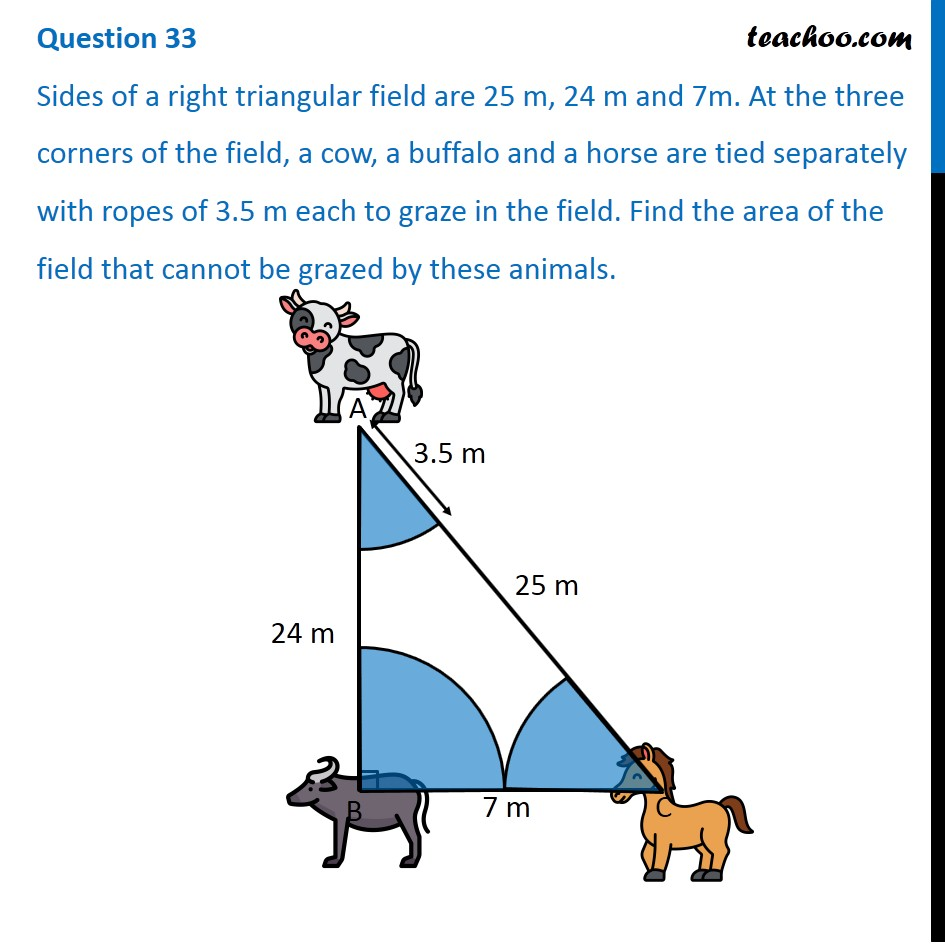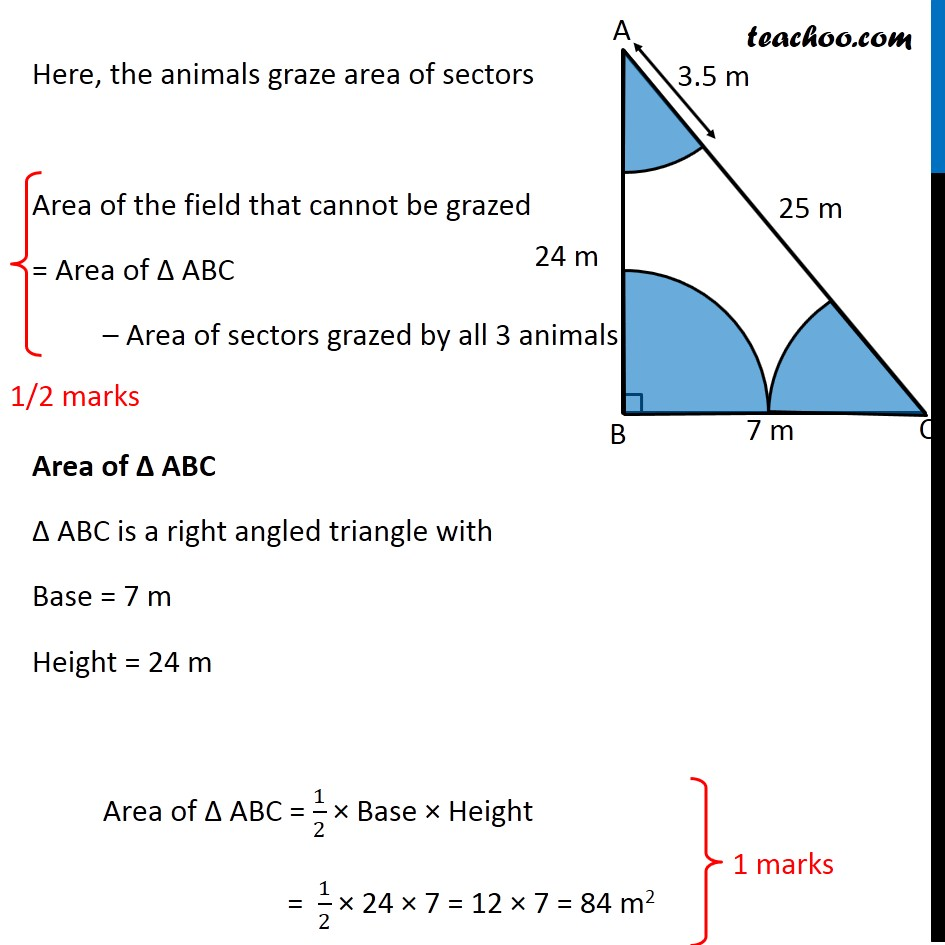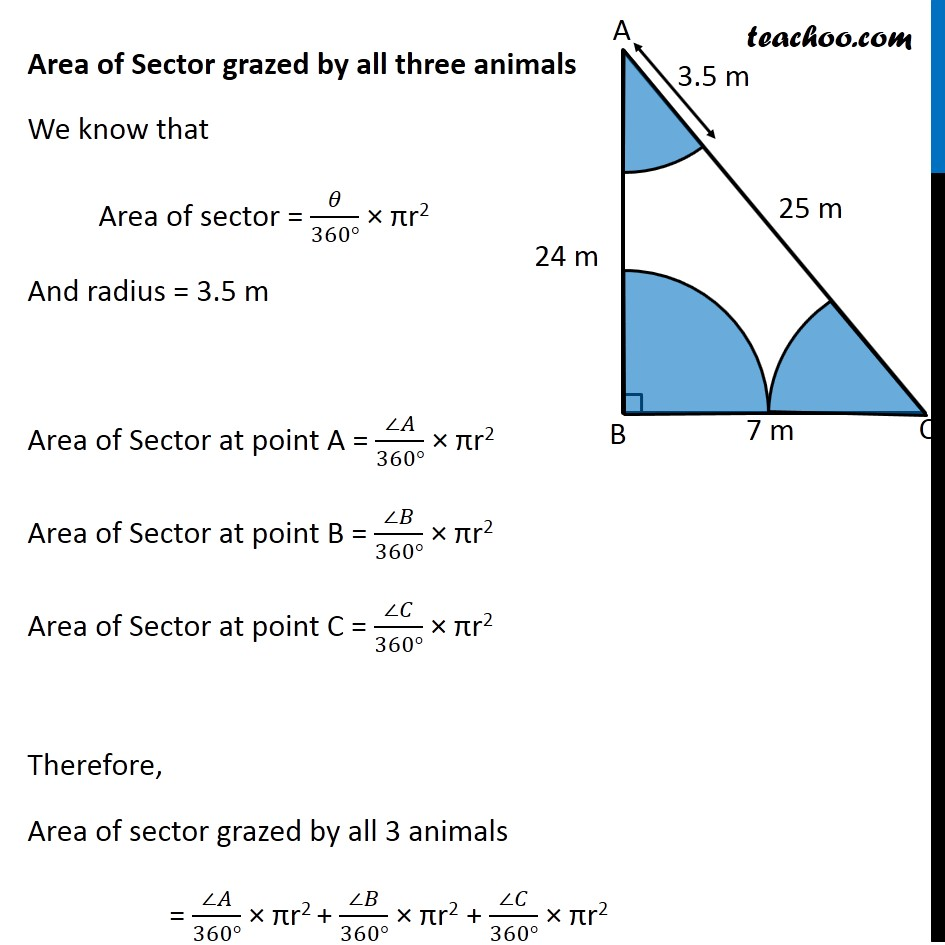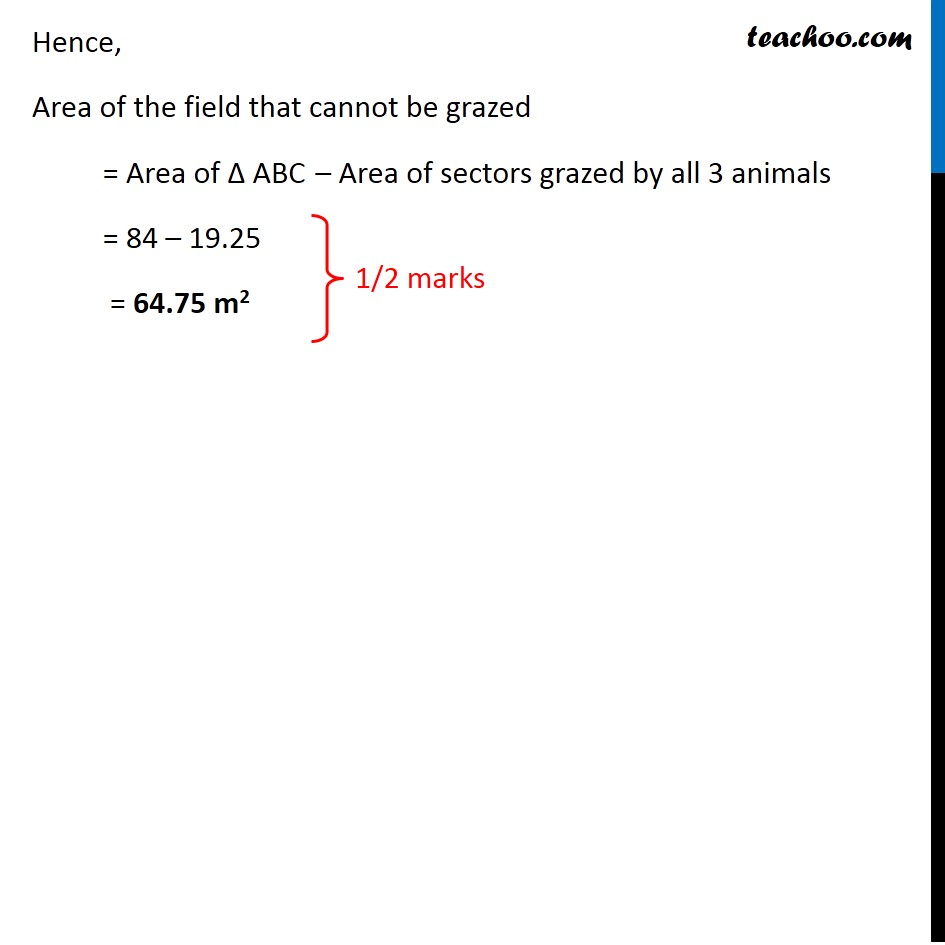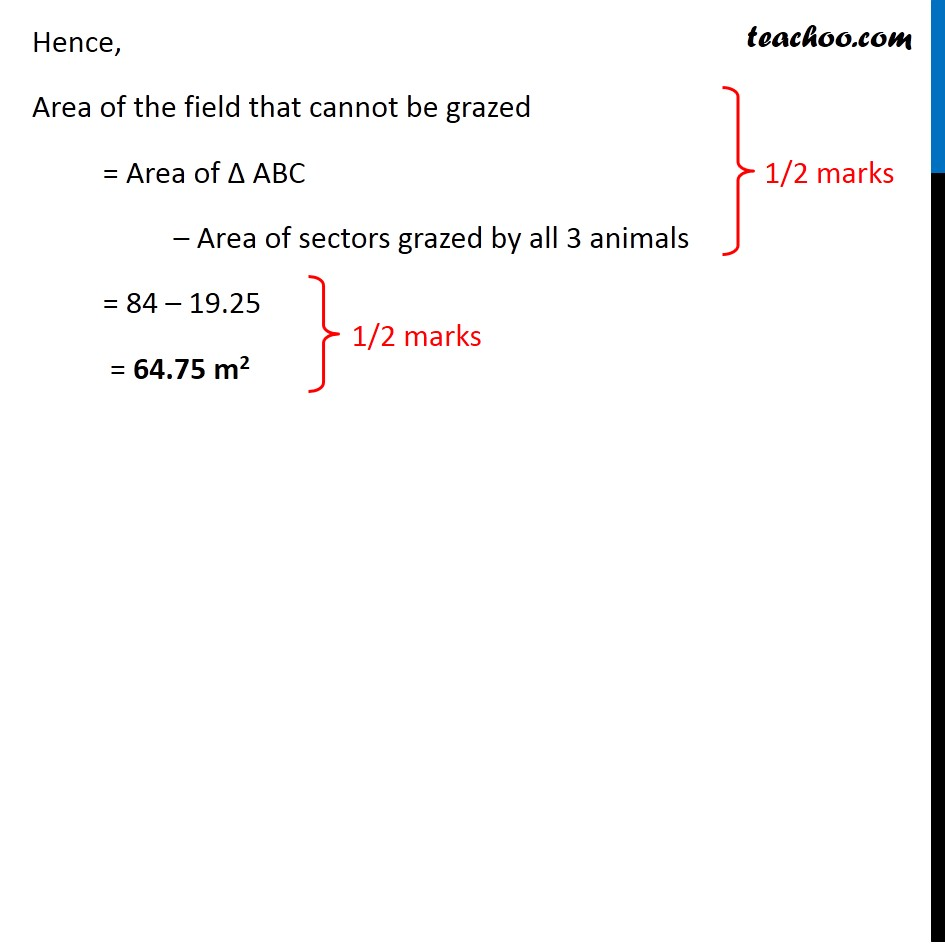Learn in your speed, with individual attention - Teachoo Maths 1-on-1 Class

### Transcript

Question 33 Sides of a right triangular field are 25 m, 24 m and 7m. At the three corners of the field, a cow, a buffalo and a horse are tied separately with ropes of 3.5 m each to graze in the field. Find the area of the field that cannot be grazed by these animals. Here, the animals graze area of sectors Area of the field that cannot be grazed = Area of Δ ABC – Area of sectors grazed by all 3 animals Area of Δ ABC Δ ABC is a right angled triangle with Base = 7 m Height = 24 m Area of Δ ABC = 1/2 × Base × Height = 1/2 × 24 × 7 = 12 × 7 = 84 m2 Area of Sector grazed by all three animals We know that Area of sector = 𝜃/(360°) × πr2 And radius = 3.5 m Area of Sector at point A = (∠𝐴)/(360°) × πr2 Area of Sector at point B = (∠𝐵)/(360°) × πr2 Area of Sector at point C = (∠𝐶)/(360°) × πr2 Therefore, Area of sector grazed by all 3 animals = (∠𝐴)/(360°) × πr2 + (∠𝐵)/(360°) × πr2 + (∠𝐶)/(360°) × πr2 = 1/(360°) × πr2 (∠ A + ∠ B + ∠ C) Since sum of angles of a triangle = 180° = 1/(360°) × πr2 × 180° = 1/2 × πr2 Putting r = 3.5 m = 1/2 × 22/7 × (3.5)2 = 11/7 × 3.5 × 3.5 = 11 × 0.5 × 3.5 = 19.25 m2 Hence, Area of the field that cannot be grazed = Area of Δ ABC – Area of sectors grazed by all 3 animals = 84 – 19.25 = 64.75 m2